Electron. J. Diff. Equ., Vol. 2014 (2014), No. 114, pp. 1-17.

### Growth of solutions to higher-order linear differential equations with entire coefficients Habib Habib, Benharrat Belaidi

Abstract:
In this article, we discuss the order and hyper-order of the linear differential equation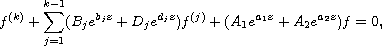where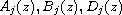are entire functions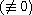and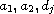are complex numbers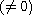, and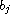are real numbers. Under certain conditions, we prove that every solutionof the above equation is of infinite order. Then, we obtain an estimate of the hyper-order. Finally, we give an estimate of the exponent of convergence for distinct zeros of the functions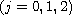, where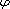is an entire functionand of order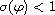, while the solution f of the differential equation is of infinite order. Our results extend the previous results due to Chen, Peng and Chen and others.

Submitted November 22, 2013. Published April 21, 2014.
Math Subject Classifications: 34M10, 30D35.
Key Words: Linear differential equation; entire solution; order of growth; hyper-order of growth; fixed point.

Show me the PDF file (312 KB), TEX file, and other files for this article.Habib Habib Department of Mathematics Laboratory of Pure and Applied Mathematics University of Mostaganem (UMAB) B. P. 227 Mostaganem, Algeria email: habibhabib2927@yahoo.fr Benharrat Belaïdi Department of Mathematics Laboratory of Pure and Applied Mathematics University of Mostaganem (UMAB) B. P. 227 Mostaganem, Algeria email: belaidi@univ-mosta.dz